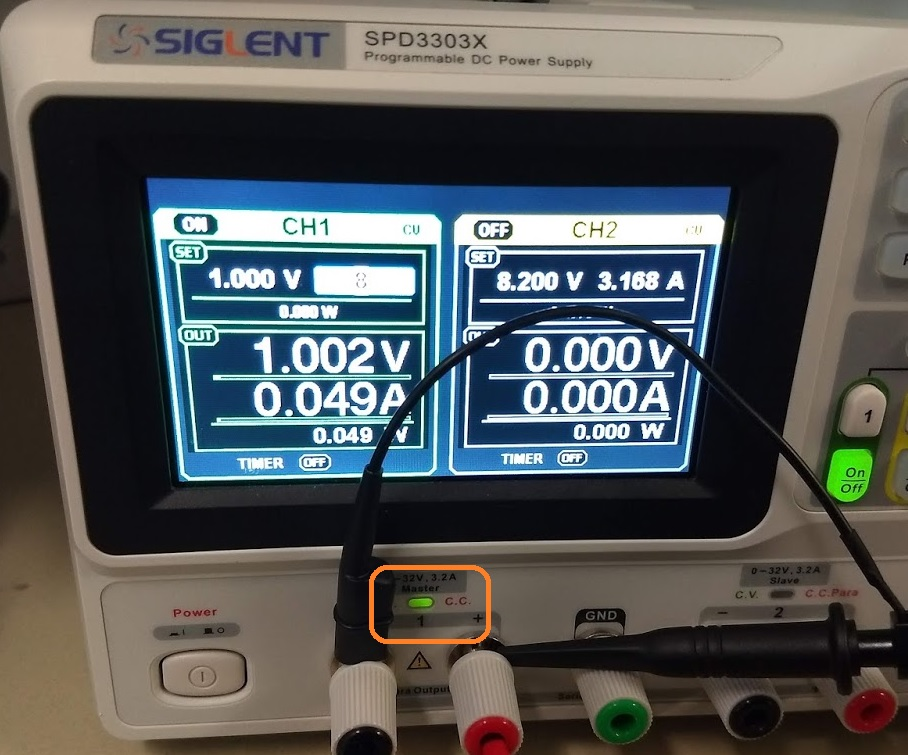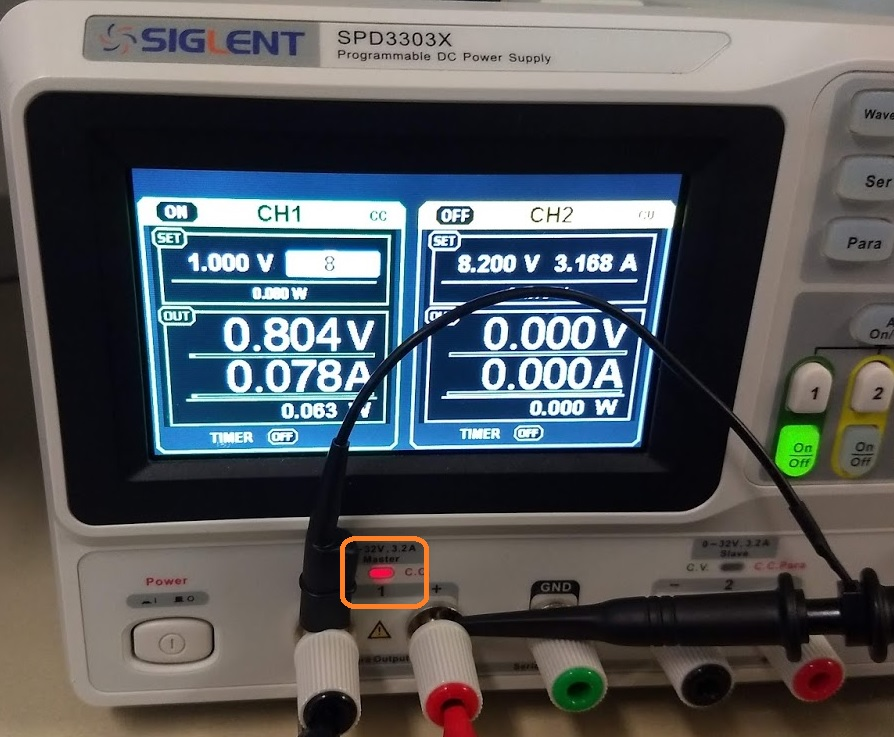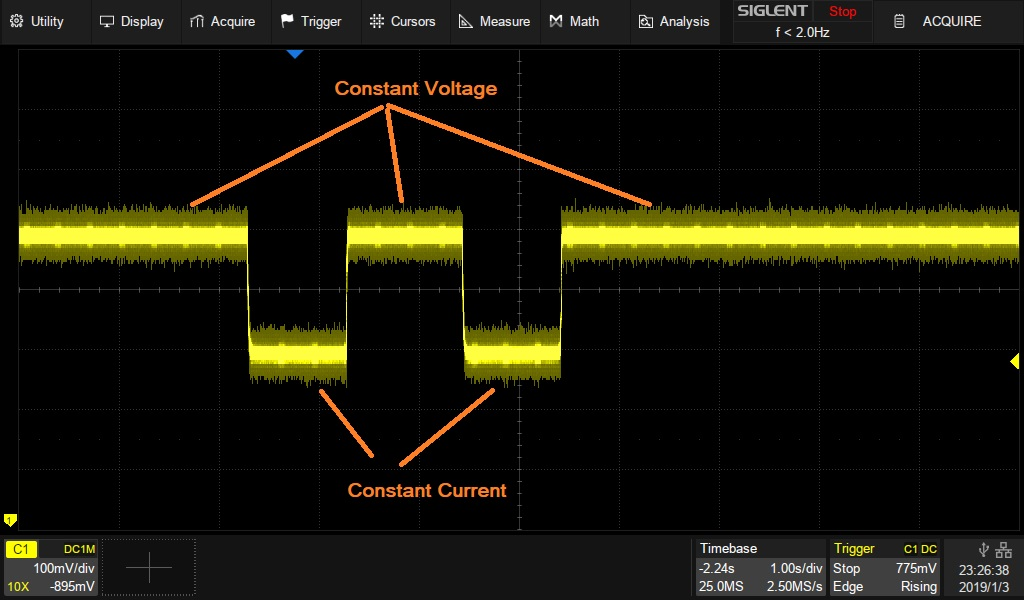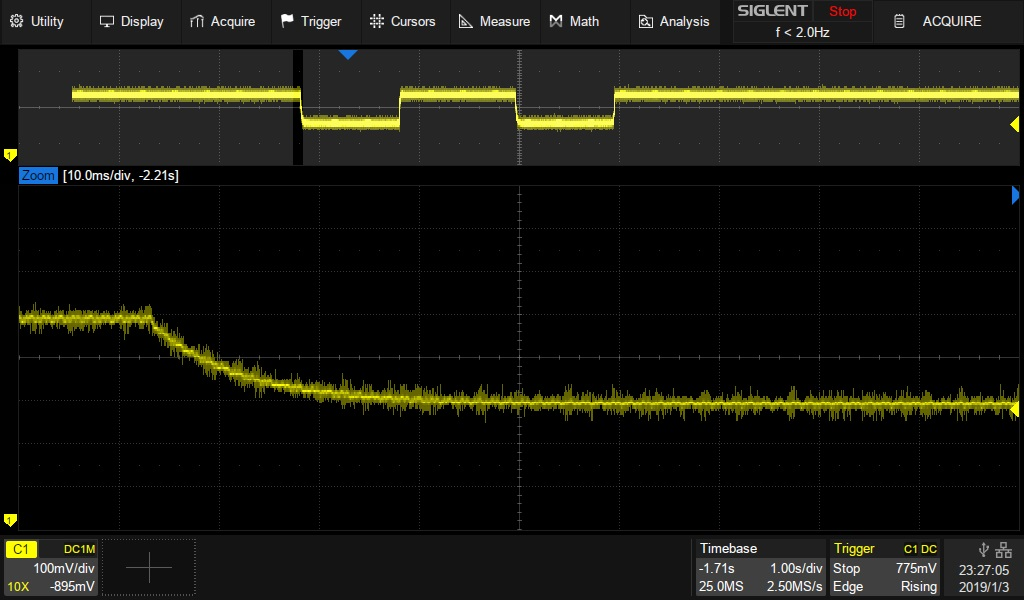# SPD constant current performance (current compliance protection)

January 3, 2019

The SIGLENT SPD series of power supplies offer constant current mode. This setting limits the current output of the specified channel and can be used as a constant current source and to protect your device-under-test (DUT).

In this tip, we show the typical performance of the SPD as it switches between constant voltage (CV) and constant current (CC) mode.

The DUT is a manually selectable resistor decade box connected to an SPD3303X power supply and an oscilloscope to capture the transient voltages as we switch between constant voltage and current.

DUT resistance = 20 Ω

SPD CH1 output set to 1 V / 0.08 A

Ohm’s law states:

V= I*R

I = V/R

So, the expected current draw is:

I = 1 V/20 Ω = 0.050 A

Here, the SPD is sourcing 1 V into 20 Ω. The measured current draw is 0.049 A and the supply is in constant voltage mode, indicated by the green CV LED on the front panel (above).Now, if we lower the resistance to 10 Ω, the calculated current draw becomes:

I = 1 V/10 Ω = 0.100 A

Now, the measured value. We can see that the current has increased and stops at just below the current setpoint of 80 mA. Here, the instrument goes into constant current mode as shown by the red LED CC indication between the output terminals:What about the voltage during this transition?

Here, we have some scope captures of the transitions from constant voltage to current:So, the transitions can be very smooth. This prevents damage to the DUT and allows you to use the SPD as a constant current source.

NOTE: This example uses a DUT with primarily resistive impedance. Circuits/DUTs with high capacitance/inductance may exhibit more pronounced output changes than shown. Minimizing extraneous impedance will help minimize glitches and spiking considerably. The main contributor is cabling (length and type).